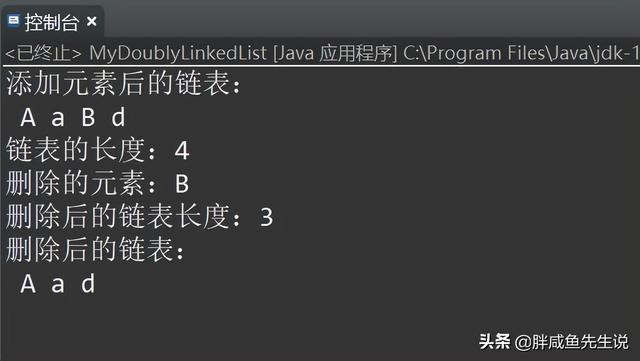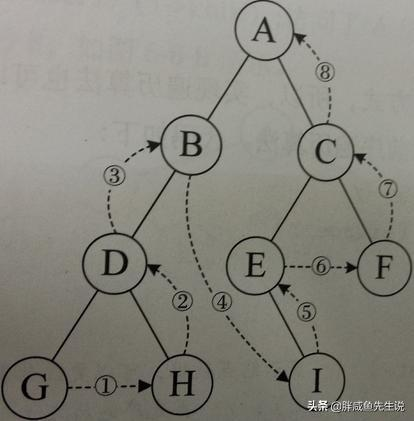### Java基础——数据结构# 1.栈结构

`package cn.pxy.test; import java.util.Arrays; import java.util.EmptyStackException; /** * 自定义栈容器 * @author 胖咸鱼 * @param <E> * */ public class MyStack<E> { private Object[] arr;//存放元素的物理结构，此时数组被定义，但未创建，使用需初始化 private int stackLength=4;//数组的默认长度 private int size;//记录栈容器的元素个数 private int index=-1;//操作数组下标位置的指针，开始时，不指向元素。 /** * 判断栈是否为空 * @return */ public boolean empty() { return this.size==0; } /** * 获取栈顶元素 * @return */ public E pop() { //如果栈容器里没有元素则抛出异常 if(this.index==-1) { throw new EmptyStackException(); } //记录元素个数，取出后栈里的元素-1 this.size--; //返回栈顶元素,指针向下移动 return (E)this.arr[index--]; } /** * 向容器中添加元素 * @param item * @return */ public E push(E item) { //初始化数组 this.capacity(); //向数组中添加元素,利用自增，把栈的指针指向添加的元素 this.arr[++index]=item; //记录元素个数 this.size++; return item; } /** * 数组初始化或以1.5倍容量对数组进行扩容 */ private void capacity() { //数组初始化 //如果数组不存在，则初始化数组，以免重复创建数组 if(this.arr==null) { this.arr=new Object[this.stackLength]; } //以1.5倍容量对数组扩容,相当于新建一个1.5倍容量的数组，把旧的数组复制进去 if(this.size-(this.stackLength-1)>=0) { this.stackLength=this.stackLength+(this.stackLength>>1); this.arr=Arrays.copyOf(this.arr, this.stackLength); } } public static void main(String[] args) { MyStack<String> myStack=new MyStack<>(); //添加元素，添加6个元素测试扩容 myStack.push("a"); myStack.push("b"); myStack.push("c"); myStack.push("d"); myStack.push("e"); myStack.push("f"); System.out.println(myStack.size); System.out.println(myStack.pop()); System.out.println(myStack.pop()); System.out.println(myStack.empty()); System.out.println(myStack.size); } }`...

# 单向链表结构...

`package cn.pxy.test; /** * 基于链表结构存储元素的方法API定义 * @author 胖咸鱼 * * @param <E> */ public interface MyList<E> { //添加元素的方法 void add(E element); //获取元素的方法 E get (int index); //获取元素个数的方法 int size(); //根据元素位置删除元素的方法 E remove(int index); }`

`package cn.pxy.test; /** * 基于单向列表实现元素存取的容器 * @author 胖咸鱼 * * @param <E> */ public class MySinglyLinkedList<E> implements MyList<E> { /** * 定义单向列表中的节点对象 */ class Node<E>{ private E item;//存储元素 private Node next;//存储下一个节点对象的地址 Node(E item,Node next){ this.item=item; this.next=next; } } private Node head;//存放链表中的头节点 private int size;//记录元素个数 /** * 向列表中添加元素 */ @Override public void add(E element) { // 创建节点 Node<E> node=new node<>(element,null); //找到尾结点 Node tail=getTail(); //节点挂接 if(tail==null) { this.head=node; }else { tail.next=node; } //记录个数 this.size++; } /** * 找到尾结点 */ private Node getTail() { //头节点是否存在 if(this.head==null) { return null; } //查找尾结点 Node node=this.head;//node指向头结点，通过移动node来找尾结点 while(true) { if(node.next==null) {//如果找到尾结点，则退出循环 break; } node=node.next;//移动指针指向下一个节点 } return node; } /** * 根据元素位置获取元素 */ @Override public E get(int index) { // 校验index的合法性 this.checkIndex(index); //根据位置获取指定节点 Node<E> node=this.getNode(index); //将该节点中的元素返回 return node.item; } /** * 对Index进行校验 */ private void checkIndex(int index) { if(!(index>=0 && index<this.size)) { throw new IndexOutOfBoundsException("Index:"+index+" Size:"+this.size); } } /** * 根据位置获取节点 */ private Node getNode(int index) { Node<E> node=this.head; for(int i=0;i<index;i++) { node=node.next; } return node; } /** * 获取元素个数 */ @Override public int size() { return this.size; } /** * 根据元素位置删除元素 */ @Override public E remove(int index) { // 校验index的合法性 this.checkIndex(index); //根据位置找到该节点的对象 Node<E> node=this.getNode(index); //获取节点对象中的元素 E item=node.item; //将该节点对象从单向列表中移除 if(this.head==node) {//判断删除的节点是否为头节点 this.head=node.next; }else { Node<E> temp=this.head; for(int i=0;i<index-1;i++) {//找到要删除节点前面的节点 temp=temp.next; } temp.next=node.next; } node.next=null; //记录元素个数 this.size--; //将该元素返回 return item; } public static void main(String[] args) { MySinglyLinkedList<String> msll=new MySinglyLinkedList<>(); msll.add("a"); msll.add("b"); msll.add("c"); msll.add("d"); System.out.println("删除前元素个数为："+msll.size); System.out.println(msll.remove(2)); System.out.println("删除后元素个数为："+msll.size); for(int i=0;i<msll.size;i++) { System.out.println(msll.get(i)); } } }`

# 双向链表结构...

`package cn.pxy.test; /** * 基于双向链表实现元素存取的容器 * @author 胖咸鱼 * */ public class MyDoublyLinkedList<E> implements MyList<E> { /** * 定义双向列表节点对象 */ class Node<E>{ E item;//记录元素 Node<E> prev;//记录前一个节点对象 Node<E> next;//记录后一个节点对象 Node(Node<E> prev,E item,Node<E> next){ this.prev=prev; this.item=item; this.next=next; } } private Node head;//记录头结点 private Node tail;//记录尾结点 private int size;//记录元素个数 /** * 添加元素,默认在尾部添加 */ @Override public void add(E element) { this.linkLast(element); } /** * 将节点对象添加到双向链表的尾部 */ private void linkLast(E element) { //获取尾结点 Node t=this.tail; //创建新节点,由于添加到尾部，所以新节点的头结点是原先链表的尾结点（t） Node<E> node=new Node<>(t,element,null); //将新节点定义为尾结点，即添加节点后，新的节点变为链表尾结点 this.tail=node; //如果链表没有元素，则新添加的节点即是尾结点又是头结点 if(t==null) { this.head=node; }else {//如果链表不为空，则原先链表的尾结点（t）的后置要指向新的节点 t.next=node; } this.size++; } /** * Index合法性的校验 */ private void checkIndex(int index) { if(!(index>=0 && index<this.size)) { throw new IndexOutOfBoundsException("Index:"+index+" Size:"+this.size); } } /** * 根据指定位置获取元素 */ @Override public E get(int index) { // 校验index this.checkIndex(index); //根据位置查找节点对象 Node<E> node=this.getNode(index); //返回节点元素 return node.item; } /** * 根据位置查找节点对象 */ private Node getNode(int index) { //判断查找的位置离头结点近，还是尾结点近 if(index<(this.size>>1)) { Node node=this.head; for(int i=0;i<index;i++) { node=node.next; } return node; }else { Node node=this.tail; for(int i=this.size-1;i>index;i--) { node=node.prev; } return node; } } /** * 返回元素个数 */ @Override public int size() { return this.size; } /** * 根据指定位置删除元素 */ @Override public E remove(int index) { // 对index进行合法性校验 this.checkIndex(index); //根据指定位置获取节点对象 Node<E> node=this.getNode(index); //获取节点对象中的元素 E item=node.item; //判断当前节点是否为头结点 if(node.prev==null) { this.head=node.next; }else { //完成当前节点的直接前驱节点与当前节点的直接后继节点的挂接 node.prev.next=node.next; } //判断当前节点是否为尾结点 if(node.next==null) { this.tail=node.prev; }else { //完成当前节点的直接后继节点与当前节点的直接前驱节点的挂接 node.next.prev=node.prev; } //当前节点断掉与他直接前驱节点的连接、断掉直接后继节点的连接 node.prev=null; node.next=null; node.item=null; //记录元素个数 this.size--; return item; } /** * 在双向链表的头部添加元素 * @param args */ public void addFirst(E element) { this.linkFirst(element); } /** * 在链表的头部添加元素 * @param element */ private void linkFirst(E element) { // 获取头结点对象 Node head=this.head; //定义新节点 Node node=new Node(null,element,head); //将新节点设为头节点 this.head=node; //判断当前链表中是否有节点，如果没有，那么该节点即使头结点，也是尾结点 if(head==null) { this.tail=node; }else { head.prev=node; } //记录元素个数 this.size++; } /** * 在链表的尾部添加元素 * @param args */ public void addLast(E element) { this.linkLast(element); } public static void main(String[] args) { MyDoublyLinkedList<String> myList=new MyDoublyLinkedList<>(); myList.add("a"); myList.addFirst("A"); myList.addLast("B"); myList.add("d"); System.out.println("添加元素后的链表："); for(int i=0;i<myList.size();i++) { System.out.print(" "+myList.get(i)); } System.out.println(); System.out.println("链表的长度："+myList.size()); System.out.println("删除的元素："+myList.remove(2)); System.out.println("删除后的链表长度："+myList.size()); System.out.println("删除后的链表："); for(int i=0;i<myList.size();i++) { System.out.print(" "+myList.get(i)); } } }`...

# 1.树形结构简介...

# 3.二叉树简介

3.1二叉树分类：......

3.2二叉树遍历：

1.前序遍历：根-左-右

2.中序遍历：左-根-右

3.后序遍历：左-右-根

4.层序遍历：从上至下逐层遍历............

3.3 二叉树排序...

`package cn.pxy.test; /** * 基于二叉树结构实现元素排序处理的排序器 * @author 胖咸鱼 * */ public class BinaryTreeSort<E extends Integer> { /** * 定义节点类 */ class Node<E extends Integer>{ private E item;//存放元素 private Node left;//存放左子树地址 private Node rigtt;//存放右子树 Node(E item){ this.item=item; } /** * 添加节点 */ public void addNode(Node node) { //完成新节点中的元素与当前节点中的元素的判断 //如果新节点中的元素小于当前节点中的元素，那么新节点放在当前节点的左子树中 if(node.item.intValue()<this.item.intValue()) { if(this.left==null) { this.left=node; }else { this.left.addNode(node); } }else { //如果新节点中的元素大于当前节点中的元素，那么新节点放在当前节点的右子树中 if(this.rigtt==null) { this.rigtt=node; }else { this.rigtt.addNode(node); } } } /** * 使用中序遍历二叉树(左-根-右) * * 递归的本质就是压栈与出栈的操作 * 当递归调用时每次调用自己时可以看做是压栈过程，当递归条件满足结束时， * 递归一级一级的返回时可以看做是出栈的过程。 * */ public void inorderTraversal() { //找到最左侧的那个节点 if(this.left!=null) { this.left.inorderTraversal(); } System.out.println(this.item); if(this.rigtt!=null) { this.rigtt.inorderTraversal(); } } } private Node root;//存放根节点 /** * 将元素添加到排序器中 */ public void add(E element) { //实例化节点对象 Node<E> node=new Node<>(element); //判断当前二叉树中是否有根节点，如果没有那么新节点为根节点 if(this.root==null) { this.root=node; }else { this.root.addNode(node); } } /** * 对元素进行排序 */ public void sort() { //判断根节点是否为空 if(this.root==null) return; this.root.inorderTraversal(); } public static void main(String[] args) { BinaryTreeSort<Integer> bts=new BinaryTreeSort<>(); bts.add(1); bts.add(8); bts.add(6); bts.add(3); bts.add(5); bts.add(2); //排序 bts.sort(); } }`

...

# 4.自定义树形结构容器

4.1树形结构定义

4.2自定义树形结构分析

...

4.3实现自定义树形结构容器

`package cn.pxy.test; import java.util.ArrayList; import java.util.HashMap; import java.util.List; import java.util.Map; /** * 基于树形结构实现元素存储的容器 * @author 胖咸鱼 * */ public class MyTree<E> { private Map<E,E> map=new HashMap<>();//String------String private Map<E,List<E>> map2=new HashMap<>();//String---List /** * 向容器中添加元素 */ public void add(E parent,E item) { //完成在单节点之间的映射 this.map.put(item, parent); //完成多节点之间映射 List<E> list=this.map2.get(parent); //判断当前节点下是否含有子节点，如果没有则创建一个新的list if(list==null) { list=new ArrayList<>(); this.map2.put(parent, list); } list.add(item); } /** * 获取当前节点的父节点 */ public E getParent(E item) { return this.map.get(item); } /** * 获取当前节点的子节点 */ public List<E> getChild(E item){ return this.map2.get(item); } /** * 获取当前节点的兄弟节点 */ public List<E> getBrother(E item){ //获取当前节点的符节点 E parent=this.getParent(item); //获取当前符节点的所有的子节点 List<E> list=this.getChild(parent); List<E> brother=new ArrayList<>(); if(list!=null) { brother.addAll(list); brother.remove(item); } return brother; } /** * 获取当前节点的祖先节点 */ public List<E> getForeFaqthers(E item){ //获取当前节点的父节点 E parent=this.getParent(item); //结束递归的边界条件 if(parent==null) { return new ArrayList<>(); } //递归调用，再次获取当前节点父节点的父节点 List<E> list=this.getForeFaqthers(parent); //将递归到的所有节点元素添加到返回的list中 list.add(parent); return list; } /** * 获取当前节点的子孙节点 */ public List<E> getGrandChildren(E item){ //存放所有子孙节点中的元素 List<E> list=new ArrayList<>(); //获取当前节点的子节点 List<E> child=this.getChild(item); //结束递归的边界条件 if(child==null) { return list; } //遍历子节点 for(int i=0;i<child.size();i++) { //获取节点中的元素 E ele=child.get(i); List<E> temp=this.getGrandChildren(ele); list.add(ele); list.addAll(temp); } return list; } public static void main(String[] args) { //实例化容器 MyTree<String> myTree=new MyTree<>(); //添加元素 myTree.add("root", "生物"); myTree.add("生物","植物"); myTree.add("生物","动物"); myTree.add("生物","菌类"); myTree.add("动物","脊椎动物"); myTree.add("动物","脊索动物"); myTree.add("动物","腔肠动物"); myTree.add("脊椎动物","哺乳动物"); myTree.add("脊椎动物","鱼类"); myTree.add("哺乳动物","猫"); myTree.add("哺乳动物","牛"); myTree.add("哺乳动物","人"); System.out.println("---------获取父结点---------"); String parent = myTree.getParent("鱼类"); System.out.println(parent); System.out.println("---------获取子结点---------"); List<String> child= myTree.getChild("动物"); for(int i=0;i<child.size();i++){ System.out.println(child.get(i)); } System.out.println("---------获取兄弟结点---------"); List<String> brother = myTree.getBrother("脊椎动物"); for(int i=0;i<brother.size();i++){ System.out.println(brother.get(i)); } System.out.println("---------获取祖先结点---------"); List<String> foreFathers = myTree.getForeFaqthers("人"); for(int i=0;i<foreFathers.size();i++){ System.out.println(foreFathers.get(i)); } System.out.println("---------获取子孙结点---------"); List<String> grandChildren = myTree.getGrandChildren("root"); for(int i =0;i<grandChildren.size();i++){ System.out.println(grandChildren.get(i)); } } }`

...